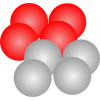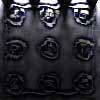#### You may also like### Fixing the Odds

You have two bags, four red balls and four white balls. You must put all the balls in the bags although you are allowed to have one bag empty. How should you distribute the balls between the two bags so as to make the probability of choosing a red ball as small as possible and what will the probability be in that case?### Scratch Cards

To win on a scratch card you have to uncover three numbers that add up to more than fifteen. What is the probability of winning a prize?### Escalator

At Holborn underground station there is a very long escalator. Two people are in a hurry and so climb the escalator as it is moving upwards, thus adding their speed to that of the moving steps. ... How many steps are there on the escalator?

# Guessing the Graph

### Why do this problem?

This problem offers learners the experience of dealing with experimental data in a scientific way, challenging them to fit a curve to some real data and deduce its equation. The task offers a real-life context for manipulating quadratic graphs and considering transformations of graphs.

As the data come from an actual experiment, there is the opportunity to make links with science lessons by suggesting learners reproduce similar experiments themselves. There is the chance for genuine cross-curricular collaboration with science teachers by offering to carry out similar tasks on data collected in the course of their science lessons.

### Possible approach

Introduce the learners to the idea that they will be given some data from a real scientific experiment, and their task will be to hypothesise about the relationship between the two variables using as few datapoints as possible.

The activity works well in pairs, so learners can discuss their ideas as they work. They will need graph paper and to draw a horizontal axis for time going from 0 to 1.6 seconds, and a vertical axis going up to 210cm.

Explain that the problem is intended to simulate the process of scientific enquiry, where scientists have to start explaining patterns from a limited amount of data and then use further experiments to confirm or reject their hypotheses. Learners can access Data Set A by shuffling and then selecting at random cards from set A

Once they have plotted a few points, they should sketch the line or curve they think best represents the data, and then try a few extra points to refine their conjecture if necessary. Learners could use different colours to represent the points they plotted and the lines or curves they drew at each stage.

Once they have drawn a curve that seems to match the data well, challenge them to work out a possible equation for it. The data is available in this Excel spreadsheet which learners could use to try out ideas and plot graphs matching the data closely. Alternatively, the class could discuss how a familiar curve such as $y=x^2$ could be transformed to resemble the curve they have sketched, leading on to work on transforming graphs by reflecting and stretching.

Data sets B and C can be used in the same way.

Take some time to discuss what the experiment could have been. The problem How Do You React? explores vertical motion under gravity, and could be attempted alongside this problem to explain the parabola shapes generated. Discussion of conservation of energy and elastic collisions may be appropriate with learners who have met these ideas in science lessons.

You may wish to recreate the experiment with your class - the data were gathered by filming the experiment and then advancing the video one frame at a time to take measurements. Of course, there are many other simple experiments into motion that could be carried out, with opportunities to predict the sorts of graphs that would result.

### Key questions

Are the points you have best suited to a straight line or a curve?
How can we transform the graph $y=x^2$ into an inverted quadratic graph?
How can we make a quadratic graph more or less curvy?
How can we move a quadratic graph up and down the y axis?
What experiment might have been done to get these results?
Why do Data Sets B and C give a shallower curve?

### Possible extension

As the experimental data represent motion of a falling object, learners could use ideas from mechanics/physics to model the situation theoretically and compare the experimental data with a theoretical value for acceleration due to gravity.

The stage 5 problem Equation Matcher offers the opportunity to consider more complicated functions passing through sets of points.

### Possible support

Use all the cards, face up, rather than selecting them randomly, and rather than focusing on the equations of the curves, spend time exploring where the data might have come from and why that shape of curve arises.